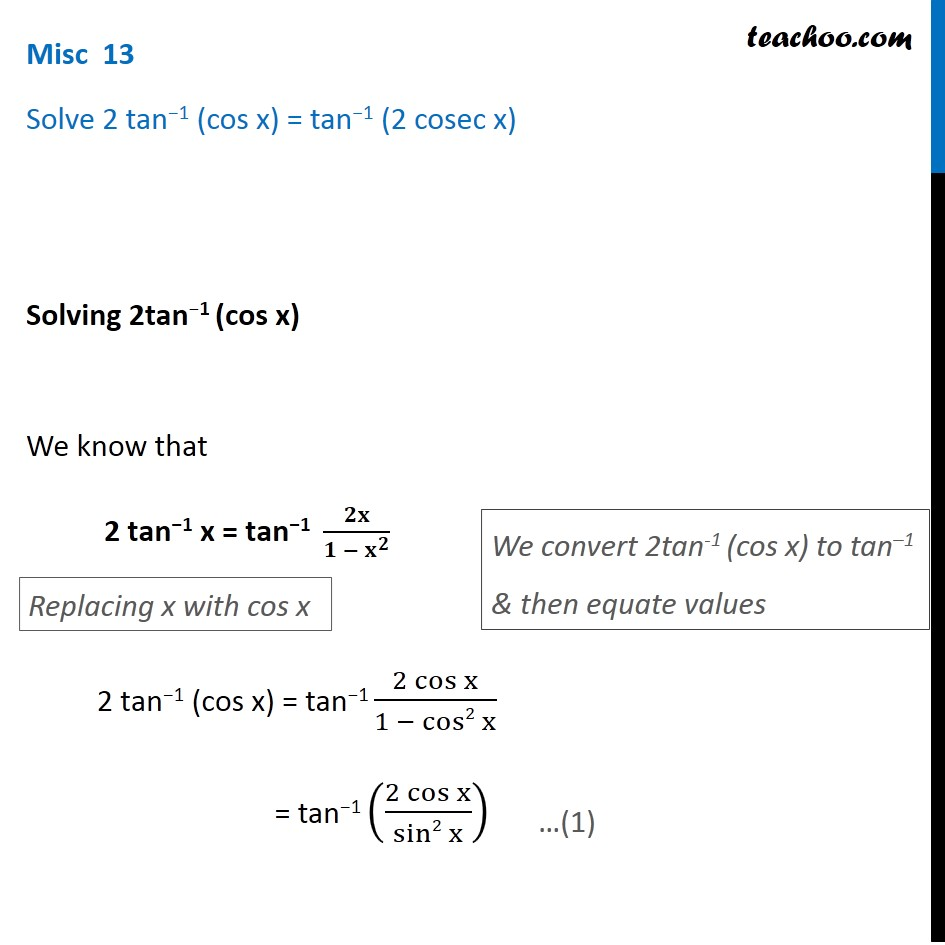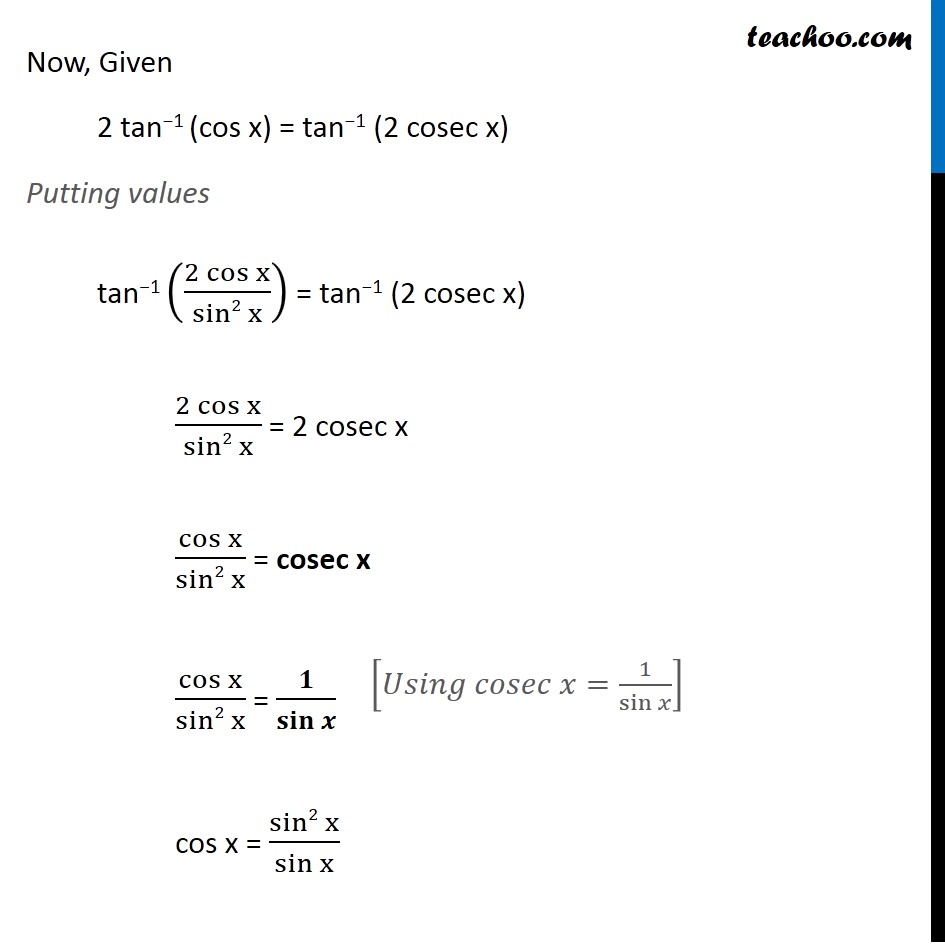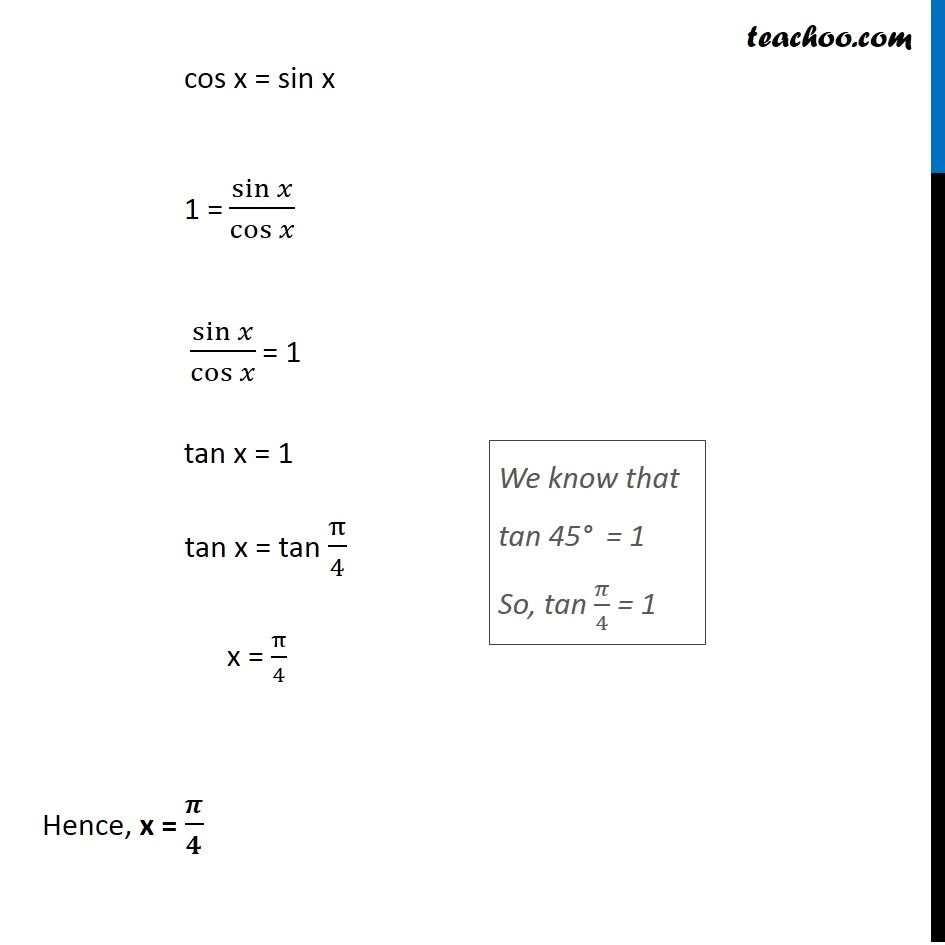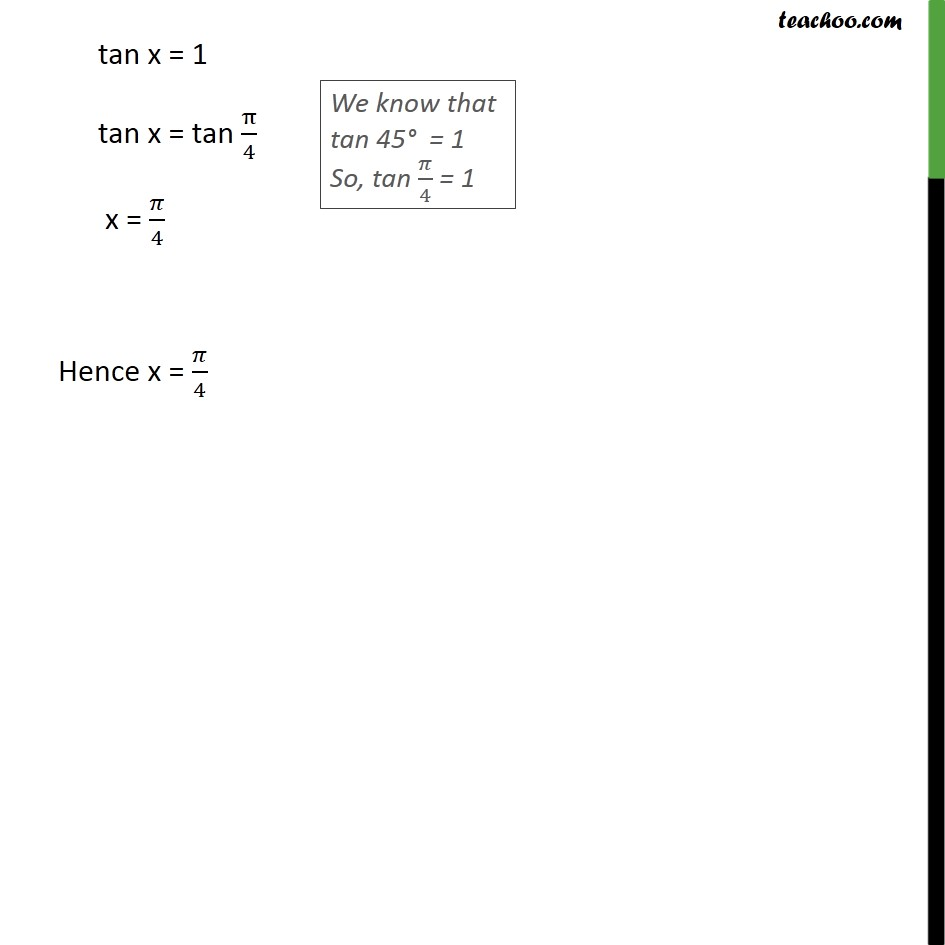Subscribe to our Youtube Channel - https://you.tube/teachoo

1. Chapter 2 Class 12 Inverse Trigonometric Functions
2. Serial order wise
3. Miscellaneous

Transcript

Misc 13 Solve 2 tan−1 (cos x) = tan−1 (2 cosec x) Solving 2tan−1 (cos x) We know that "2 tan−1 x = tan−1 " 𝟐𝐱/(𝟏 − 𝐱^𝟐 ) "2 tan−1 (cos x)" = tan−1 (2 cos⁡x)/(1 − cos2 x) = tan−1 ((2 cos⁡x)/(sin2 x)) Replacing x with cos x We convert 2tan-1 (cos x) to tan–1 & then equate values Now, Given 2 tan−1 (cos x) = tan−1 (2 cosec x) Putting values tan−1 ((2 cos⁡x)/(sin2 x)) = tan−1 (2 cosec x) (2 cos⁡x)/(sin2 x) = 2 cosec x cos⁡x/(sin2 x) = cosec x cos⁡x/(sin2 x) = 𝟏/(𝐬𝐢𝐧 𝒙) cos x = (sin2 x)/(sin x) [𝑈𝑠𝑖𝑛𝑔 𝑐𝑜𝑠𝑒𝑐 𝑥=1/sin⁡𝑥 ] cos x = sin x 1 = sin⁡𝑥/cos⁡𝑥 sin⁡𝑥/cos⁡𝑥 = 1 tan x = 1 tan x = tan π/4 x = π/4 Hence, x = 𝝅/𝟒 We know that tan 45° = 1 So, tan 𝜋/4 = 1

Miscellaneous